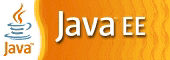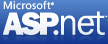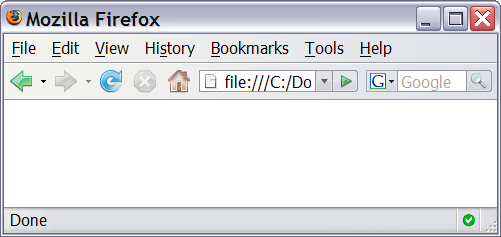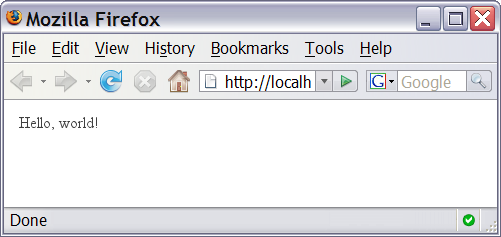# Web Programming Step by Step

### Chapter 5 PHP for Server-Side Programming

Except where otherwise noted, the contents of this presentation are Copyright 2009 Marty Stepp and Jessica Miller.

# 5.1: Server-Side Basics

• 5.1: Server-Side Basics
• 5.2: PHP Basic Syntax
• 5.3: Embedded PHP

# URLs and web servers

```http://server/path/file
```
• usually when you type a URL in your browser:
• your browser connects to that IP address and requests the given file
• the web server software (e.g. Apache) grabs that file from the server's local file system, and sends back its contents to you
• some URLs actually specify programs that the web server should run, and then send their output back to you as the result:
```https://webster.cs.washington.edu/quote2.php
```
• the above URL tells the server `webster.cs.washington.edu` to run the program `quote2.php` and send back its output

# Server-Side web programming• server-side pages are programs written using one of many web programming languages/frameworks
• the web server contains software that allows it to run those programs and send back their output as responses to web requests
• each language/framework has its pros and cons
• we use PHP for server-side programming in this textbook

# What is PHP? (5.1.2)• PHP stands for "PHP Hypertext Preprocessor"
• a server-side scripting language
• used to make web pages dynamic:
• provide different content depending on context
• interface with other services: database, e-mail, etc
• authenticate users
• process form information
• PHP code can be embedded in XHTML code

# Lifecycle of a PHP web request (5.1.1)• browser requests a `.html` file (static content): server just sends that file
• browser requests a `.php` file (dynamic content): server reads it, runs any script code inside it, then sends result across the network
• script produces output that becomes the response sent back

# Why PHP?

There are many other options for server-side languages: Ruby on Rails, JSP, ASP.NET, etc. Why choose PHP?

• free and open source: anyone can run a PHP-enabled server free of charge
• compatible: supported by most popular web servers
• simple: lots of built-in functionality; familiar syntax
• available: installed on UW's servers (Dante, Webster) and most commercial web hosts

# Hello, World!

The following contents could go into a file `hello.php`:

```<?php
print "Hello, world!";
?>
```
Hello, world!
• a block or file of PHP code begins with `<?php` and ends with `?>`
• PHP statements, function declarations, etc. appear between these endpoints

# Viewing PHP output• you can't view your `.php` page on your local hard drive; you'll either see nothing or see the PHP source code
• if you upload the file to a PHP-enabled web server, requesting the `.php` file will run the program and send you back its output

# 5.2: PHP Basic Syntax

• 5.1: Server-Side Basics
• 5.2: PHP Basic Syntax
• 5.3: Embedded PHP

# Console output: `print`(5.2.2)

```print "text";
```
```print "Hello, World!\n";
print "Escape \"chars\" are the SAME as in Java!\n";

print "You can have
line breaks in a string.";

print 'A string can use "single-quotes".  It\'s cool!';
```
Hello, World! Escape "chars" are the SAME as in Java! You can have line breaks in a string. A string can use "single-quotes". It's cool!
• some PHP programmers use the equivalent `echo` instead of `print`

# Variables(5.2.5)

```\$name = expression;
```
```\$user_name = "PinkHeartLuvr78";
\$age = 16;
\$drinking_age = \$age + 5;
\$this_class_rocks = TRUE;
```
• names are case sensitive; separate multiple words with _
• names always begin with `\$`, on both declaration and usage
• always implicitly declared by assignment (type is not written)
• a loosely typed language (like JavaScript or Python)

# Operators(5.2.4)

• ``` + - * / % . ++ -- = += -= *= /= %= .= == != === !== > < >= <= && || ! ```
• `==` just checks value (`"5.0" == 5` is `TRUE`)
• `===` also checks type (`"5" === 5` is `FALSE`)
• many operators auto-convert types: `5 < "7"` is `TRUE`

# `int` and `float` types

```\$a = 7 / 2;               # float: 3.5
\$b = (int) \$a;            # int: 3
\$c = round(\$a);           # float: 4.0
\$d = "123";               # string: "123"
\$e = (int) \$d;            # int: 123
```
• `int` for integers and `float` for reals
• division between two `int` values can produce a `float`

# Math operations

```\$a = 3;
\$b = 4;
\$c = sqrt(pow(\$a, 2) + pow(\$b, 2));
```
 `abs` `ceil` `cos` `floor` `log` `log10` `max` `min` `pow` `rand` `round` `sin` `sqrt` `tan`
 `M_PI` `M_E` `M_LN2`
• the syntax for method calls, parameters, returns is the same as Java

```# single-line comment

// single-line comment

/*
multi-line comment
*/
```
• like Java, but `#` is also allowed
• a lot of PHP code uses `#` comments instead of `//`
• we recommend `#` and will use it in our examples

# `String` type (5.2.6)

```\$favorite_food = "Ethiopian";
print \$favorite_food;            # h
```
• zero-based indexing using bracket notation
• string concatenation operator is `.` (period), not `+`
• `5 + "2 turtle doves" === 7`
• `5 . "2 turtle doves" === "52 turtle doves"`
• can be specified with `""` or `''`

# `String` functions

```\$name = "Kenneth Kuan";
\$length = strlen(\$name);              # 12
\$cmp = strcmp(\$name, "Jeff Prouty");  # > 0
\$index = strpos(\$name, "e");          # 1
\$first = substr(\$name, 8, 4);         # "Kuan"
\$name = strtoupper(\$name);            # "KENNETH KUAN"
```
NameJava Equivalent
`explode`, `implode` `split`, `join`
`strlen` `length`
`strcmp` `compareTo`
`strpos` `indexOf`
`substr` `substring`
`strtolower`, `strtoupper` `toLowerCase`, `toUpperCase`
`trim` `trim`

# Interpreted strings

```\$age = 16;
print "You are " . \$age . " years old.\n";
print "You are \$age years old.\n";    # You are 16 years old.
```
• strings inside `" "` are interpreted
• variables that appear inside them will have their values inserted into the string
• strings inside `' '` are not interpreted:
```print 'You are \$age years old.\n';    # You are \$age years old.\n
```
• if necessary to avoid ambiguity, can enclose variable in `{}`:
```print "Today is your \$ageth birthday.\n";     # \$ageth not found
print "Today is your {\$age}th birthday.\n";
```

# `for` loop (same as Java)(5.2.9)

```for (initialization; condition; update) {
statements;
}
```
```for (\$i = 0; \$i < 10; \$i++) {
print "\$i squared is " . \$i * \$i . ".\n";
}
```

# bool (Boolean) type (5.2.8)

```\$feels_like_summer = FALSE;

\$student_count = 217;
\$nonzero = (bool) \$student_count;     # TRUE
```
• the following values are considered to be `FALSE` (all others are `TRUE`):
• `0` and `0.0` (but NOT `0.00` or `0.000`)
• `""`, `"0"`, and `NULL` (includes unset variables)
• arrays with 0 elements
• can cast to boolean using `(bool)`
• `FALSE` prints as an empty string (no output); `TRUE` prints as a `1`
• `TRUE` and `FALSE` keywords are case insensitive

# `if/else` statement

```if (condition) {
statements;
} elseif (condition) {
statements;
} else {
statements;
}
```
• NOTE: although `elseif` keyword is much more common, `else if` is also supported

# `while` loop (same as Java)

```while (condition) {
statements;
}
```
```do {
statements;
} while (condition);
```
• `break` and `continue` keywords also behave as in Java

# `NULL`

```\$name = "Victoria";
\$name = NULL;
if (isset(\$name)) {
print "This line isn't going to be reached.\n";
}
```
• a variable is `NULL` if
• it has not been set to any value (undefined variables)
• it has been assigned the constant `NULL`
• it has been deleted using the `unset` function
• can test if a variable is `NULL` using the `isset` function
• `NULL` prints as an empty string (no output)

# 5.3: Embedded PHP

• 5.1: Server-Side Basics
• 5.2: PHP Basic Syntax
• 5.3: Embedded PHP

# Embedding code in web pages

• most PHP programs actually produce HTML as their output
• dynamic pages; responses to HTML form submissions; etc.
• an embedded PHP program is a file that contains a mixture of HTML and PHP code# A bad way to produce HTML in PHP

```<?php
print "<!DOCTYPE html PUBLIC \"-//W3C//DTD XHTML 1.1//EN\"\n";
print " \"http://www.w3.org/TR/xhtml11/DTD/xhtml11.dtd\">\n";
print "<html xmlns=\"http://www.w3.org/1999/xhtml\">\n";
print "    <title>My web page</title>\n";
...
?>
```
• printing HTML code with `print` statements is ugly and error-prone:
• must quote the HTML and escape special characters, e.g. `\"`
• must insert manual `\n` line breaks after each line
• don't `print` HTML; it's bad style!

# Syntax for embedded PHP (5.3.1)

```HTML content

<?php
PHP code
?>

HTML content
```
• any contents of a `.php` file that are not between `<?php` and `?>` are output as pure HTML
• can switch back and forth between HTML and PHP "modes"

# Embedded PHP example

```<!DOCTYPE html PUBLIC "-//W3C//DTD XHTML 1.1//EN"
"http://www.w3.org/TR/xhtml11/DTD/xhtml11.dtd">
<html xmlns="http://www.w3.org/1999/xhtml">
<body>
<h1>Geneva's Counting Page</h1>
<p>Watch how high I can count:
<?php
for (\$i = 1; \$i <= 10; \$i++) {
print "\$i\n";
}
?>
</p>
</body>
</html>
```
• the above code would be saved into a file such as `count.php`
• How many lines of numbers will appear? (View Source!)

# Embedded PHP + `print` = bad

```...
<h1>Geneva's Counting Page</h1>
<p>Watch how high I can count:
<?php
for (\$i = 1; \$i <= 10; \$i++) {
print "\$i\n";
}
?>
</p>
```
• best PHP style is to use as few `print`/`echo` statements as possible in embedded PHP code
• but without `print`, how do we insert dynamic content into the page?

# PHP expression blocks (5.3.2)

```<?= expression ?>
```
```<h2>The answer is <?= 6 * 7 ?></h2>
```

• PHP expression block: a small piece of PHP that evaluates and embeds an expression's value into HTML
• `<?= expression ?>` is equivalent to:
```<?php print expression; ?>
```
• useful for embedding a small amount of PHP (a variable's or expression's value) in a large block of HTML without having to switch to "PHP-mode"

# Expression block example

```<!DOCTYPE html PUBLIC "-//W3C//DTD XHTML 1.1//EN"
"http://www.w3.org/TR/xhtml11/DTD/xhtml11.dtd">
<html xmlns="http://www.w3.org/1999/xhtml">
<body>
<?php
for (\$i = 99; \$i >= 1; \$i--) {
?>
<p><?= \$i ?> bottles of beer on the wall, <br />
<?= \$i ?> bottles of beer. <br />
Take one down, pass it around, <br />
<?= \$i - 1 ?> bottles of beer on the wall.</p>
<?php
}
?>
</body>
</html>
```

# Common error: unclosed braces

```...
<body>
<p>Watch how high I can count:
<?php
for (\$i = 1; \$i <= 10; \$i++) {
?>
<?= \$i ?>
</p>
</body>
</html>
```
• if you open a `{` brace, you must have a matching `}` brace later
• `</body>` and `</html>` above are inside the `for` loop, which is never closed
• if you forget to close your braces, you'll see an error about 'unexpected `\$end`'

# Common error fixed

```...
<body>
<p>Watch how high I can count:
<?php
for (\$i = 1; \$i <= 10; \$i++) {     # PHP mode
?>
<?= \$i ?>                     <!-- HTML mode -->
<?php
}                                  # PHP mode
?>
</p>
</body>
</html>
```

# Common error: Missing `=` sign

```...
<body>
<p>Watch how high I can count:
<?php
for (\$i = 1; \$i <= 10; \$i++) {
?>
<? \$i ?>
<?php
}
?>
</p>
</body>
</html>
```
• a block between `<?` ... `?>` is often interpreted the same as one between `<?php` ... `?>`
• PHP evaluates the code, but `\$i` does not produce any output

# Complex expression blocks

```...
<body>
<?php
for (\$i = 1; \$i <= 3; \$i++) {
?>
<h<?= \$i ?>>This is a level <?= \$i ?> heading.</h<?= \$i ?>>
<?php
}
?>
</body>
```

# This is a level 1 heading.

## This is a level 2 heading.

### This is a level 3 heading.

• expression blocks can even go inside HTML tags and attributes

• 5.1: Server-Side Basics
• 5.2: PHP Basic Syntax
• 5.3: Embedded PHP

# Functions (5.4.1)

```function name(parameterName, ..., parameterName) {
statements;
}
```
```function quadratic(\$a, \$b, \$c) {
return -\$b + sqrt(\$b * \$b - 4 * \$a * \$c) / (2 * \$a);
}
```
• parameter types and return types are not written

# Calling functions

```name(parameterValue, ..., parameterValue);
```
```\$x = -2;
\$a = 3;
\$root = quadratic(1, \$x, \$a - 2);
```
• if the wrong number of parameters are passed, it's an error

# Default parameter values

```function name(parameterName, ..., parameterName) {
statements;
}
```
```function print_separated(\$str, \$separator = ", ") {
if (strlen(\$str) > 0) {
print \$str;
for (\$i = 1; \$i < strlen(\$str); \$i++) {
print \$sep . \$str[\$i];
}
}
}
```
```print_separated("hello");        # h, e, l, l, o
print_separated("hello", "-");   # h-e-l-l-o
```
• if no value is passed, the default will be used (defaults must come last)

# Variable scope: global and local vars

```\$school = "UW";                   # global
...

global \$school;
\$suffix = "Tacoma";             # local

\$school = "\$school \$suffix";
print "\$school\n";
}
```
• variables declared in a function are local to that function
• variables not declared in a function are global
• if a function wants to use a global variable, it must have a `global` statement

# Including files: `include()`(5.4.2)

```include("filename");
```
```include("header.php");
```
• inserts the entire contents of the given file into the PHP script's output page
• encourages modularity
• useful for defining reused functions like form-checking

# Arrays(5.4.3)

```\$name = array();                         # create
\$name = array(value0, value1, ..., valueN);

\$name[index]                              # get element value
\$name[index] = value;                      # set element value
\$name[] = value;                          # append
```
```\$a = array();     # empty array (length 0)
\$a = 23;       # stores 23 at index 0 (length 1)
\$a2 = array("some", "strings", "in", "an", "array");
\$a2[] = "Ooh!";   # add string to end (at index 5)
```
• to append, use bracket notation without specifying an index
• element type is not specified; can mix types

# Array functions

function name(s) description
`count` number of elements in the array
`print_r` print array's contents
`array_pop`, `array_push`,
`array_shift`, `array_unshift`
using array as a stack/queue
`in_array`, `array_search`, `array_reverse`,
`sort`, `rsort`, `shuffle`
searching and reordering
`array_fill`, `array_merge`, `array_intersect`,
`array_diff`, `array_slice`, `range`
creating, filling, filtering
`array_sum`, `array_product`, `array_unique`,
`array_filter`, `array_reduce`
processing elements

# Array function example

```\$tas = array("MD", "BH", "KK", "HM", "JP");
for (\$i = 0; \$i < count(\$tas); \$i++) {
\$tas[\$i] = strtolower(\$tas[\$i]);
}                                 # ("md", "bh", "kk", "hm", "jp")
\$morgan = array_shift(\$tas);      # ("bh", "kk", "hm", "jp")
array_pop(\$tas);                  # ("bh", "kk", "hm")
array_push(\$tas, "ms");           # ("bh", "kk", "hm", "ms")
array_reverse(\$tas);              # ("ms", "hm", "kk", "bh")
sort(\$tas);                       # ("bh", "hm", "kk", "ms")
\$best = array_slice(\$tas, 1, 2);  # ("hm", "kk")
```
• the array in PHP replaces many other collections in Java
• list, stack, queue, set, map, ...

# The `foreach` loop (5.4.4)

```foreach (\$array as \$variableName) {
...
}
```
```\$stooges = array("Larry", "Moe", "Curly", "Shemp");
for (\$i = 0; \$i < count(\$stooges); \$i++) {
print "Moe slaps {\$stooges[\$i]}\n";
}
foreach (\$stooges as \$stooge) {
print "Moe slaps \$stooge\n";  # even himself!
}
```
• a convenient way to loop over each element of an array without indexes

# Splitting/joining strings

```\$array = explode(delimiter, string);
\$string = implode(delimiter, array);
```
```\$s  = "CSE 190 M";
\$a  = explode(" ", \$s);     # ("CSE", "190", "M")
\$s2 = implode("...", \$a);   # "CSE...190...M"
```
• `explode` and `implode` convert between strings and arrays
• for more complex string splitting, we'll use regular expressions (later)

# Unpacking an array: `list`

```list(\$var1, ..., \$varN) = array;
```
```\$line = "stepp:17:m:94";
list(\$username, \$age, \$gender, \$iq) = explode(":", \$line);
```
• the `list` function accepts a comma-separated list of variable names as parameters
• assign an array (or the result of a function that returns an array) to store that array's contents into the variables

# Non-consecutive arrays

```\$autobots = array("Optimus", "Bumblebee", "Grimlock");
\$autobots = "Hotrod";
```
• the indexes in an array do not need to be consecutive
• the above array has a `count` of 4, with 97 blank elements between `"Grimlock"` and `"Hotrod"`

# PHP file I/O functions (5.4.5)

```\$text = file_get_contents("schedule.txt");
\$lines = explode("\n", \$text);
\$lines = array_reverse(\$lines);
\$text = implode("\n", \$lines);
file_put_contents("schedule.txt", \$text);
```

```# Returns how many lines in this file are empty or just spaces.
function count_blank_lines(\$file_name) {
\$text = file_get_contents(\$file_name);
\$lines = explode("\n", \$text);
\$count = 0;
foreach (\$lines as \$line) {
if (strlen(trim(\$line)) == 0) {
\$count++;
}
}
return \$count;
}
...
print count_blank_lines("ch05-php.html");
```

```\$folder = "images";
• `scandir` returns an array of all files in a given directory
• annoyingly, the current directory (`"."`) and parent directory (`".."`) are included in the array; you probably want to skip them